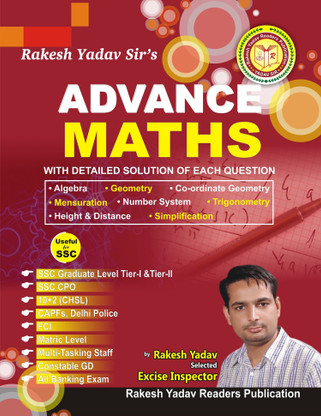• Indices and Surds
• Linear equations in two variables
• Graphic representation of straight lines
• Co-ordinate geometry
• Polynomials
• Mensuration 1 ( Area and peremeter (2D))
• Mensuration 2 ( Area and volume (3D))
• Algebric identities
• Line
• Triangles
• Center of triangles
• Triangles, Congruence and Similarity
• Circles, Chords and Tangents
• Trigonometric ratios
• Trigonometric identities
• Maximum and minimum value of trigonometric functions
• Trigonometry, Circular measurment of angles
• Height & Distance
Tags: UPSC